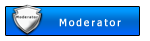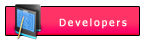affiliate marketinganyone?? master the c++.. nid help ...plssss
Convert your Points Into LOAD click here
Top posters

### Latest topics

You are not connected. Please login or register

#### Zaih27Moderatornid elp guys

#### xtrojanDeveloper[You must be registered and logged in to see this link.] wrote:nid elp guys
anong clasing C++ problem mo tol??

#### Zaih27Moderatorito ung code pre...

Code:
`#include <stdio.h>#include <string.h>#include <conio.h>#include <ctype.h>void main () {  char strSENT,strWORD,strEdit,strRep;  char chInkey,QUIT;  char TRUE = 1,EndStr;  char FALSE = 0;  int numWords,A,B,C,Z,Y,POS;  /*Ask for user's Sentence*/  lowvideo ();  printf ("\nEnter Sentence: ");  /*Usually, strSENT is an array of 80 characters */  /*An array of characters is a string*/  gets (strSENT);  /*Menu*/  QUIT = FALSE;  do  {    /*Initialize Elements of strWORD*/    for (Z=0;Z<25;Z++)    for (Y=0;Y<15;Y++)    strWORD[Z][Y] = '\0';    numWords=0;  EndStr=FALSE;  A = 0,B=0,C=0;  /*Evaluate Words*/  /*Assign Words to seperate arrays*/      do      {    /**/    if (strSENT[A]=='.')    {      strWORD[B][C]=strSENT[A];      C++;      strWORD[B][C]='\0';      B++;      C=0;    }    else if (strSENT[A]==',')    {      strWORD[B][C]=strSENT[A];      C++;      strWORD[B][C]='\0';      B++;      C=0;    }    else if (isalpha(strSENT[A]))    {      strWORD[B][C]=strSENT[A];      C++;    }    else if ((isspace(strSENT[A])) && (A>0))    {      C++;      strWORD[B][C]='\0';      B++;      C=0;    }    A++;    if (strSENT[A]=='\0') EndStr = TRUE;      }      while (EndStr==FALSE);  if (strWORD=='\0')  numWords = 1;  else numWords = B;  printf ("Your sentence is: %s\n",strSENT);  printf ("Press 1 to Insert word\n");  printf ("Press 2 to Delete word\n");  printf ("Press 3 to Edit/Replace word\n");  printf ("Press 4 to Exit\n");  printf ("Your Response: ");  chInkey=getche ();  printf ("\n");  if (chInkey == '4') QUIT = TRUE;  if (chInkey == '3')    {/*Edit/Replace Words*/      printf ("What word to replace?: ");      gets (strEdit);      printf ("Replace with what?: ");      gets (strRep);      if (strstr(strSENT,strEdit))      {    for (Z=0;Z<=numWords;Z++)    if ((strcmpi(strEdit,strWORD[Z]))==0)      /*if word matches*/      {        strcpy (strWORD[Z],strRep);      }        strcpy (strSENT,strWORD);    for (Z=1;Z<=numWords;Z++)        {        strcat (strSENT," ");        strcat (strSENT,strWORD[Z]);        }      }      else      {    printf ("Word not present in the sentence!\n");      }    }  if (chInkey == '2')    /**/    {      printf ("What word to delete?: ");      gets (strEdit);      if (strstr(strSENT,strEdit))      {    for (Z=0;Z<=numWords;Z++)    if ((strcmpi(strEdit,strWORD[Z]))==0)      /*if word matches*/      {        strWORD[Z]='\0';      }        strcpy (strSENT,strWORD);    for (Z=1;Z<=numWords;Z++)        {        strcat (strSENT," ");        strcat (strSENT,strWORD[Z]);        }      }      else      printf ("Word not found in sentence!\n");    }  if (chInkey == '1')    {    printf ("What word to insert?: ");    gets (strEdit);    printf ("Where to insert?[position no.]: ");    scanf ("%d",&POS);    strSENT='\0';    for (Z=0;Z<POS;Z++)      {    strcat (strSENT," ");    strcat (strSENT,strWORD[Z]);      }    strcat (strSENT," ");    strcat (strSENT,strEdit);    for (Y=Z;Y<=numWords;Y++)      {    strcat (strSENT," ");    strcat (strSENT,strWORD[Y]);      }    }  }  while (QUIT == FALSE); printf ("Goodbye!\n"); }`

ang gusto kong palabasin eh.. ung edit agad... delete na ung add tska delete string... tapos... panu ko magagawang ung...ni replace ko eh maticna papatunganungfirst input ko..

tska pa explain naman ng literal ung mga int variables.. di ko cla gets eh :D tnx

#### Sponsored content#### Advertising posterPermissions in this forum: You cannot reply to topics in this forum